# Design of Eccentrically Loaded Bolted/Riveted Joints Notes | Study Design of Machine Elements - Mechanical Engineering

## Mechanical Engineering: Design of Eccentrically Loaded Bolted/Riveted Joints Notes | Study Design of Machine Elements - Mechanical Engineering

The document Design of Eccentrically Loaded Bolted/Riveted Joints Notes | Study Design of Machine Elements - Mechanical Engineering is a part of the Mechanical Engineering Course Design of Machine Elements.
All you need of Mechanical Engineering at this link: Mechanical Engineering

Consider a bracket fixed to the wall by means of three rows of screws having two in each row as shown in figure 11.1.1. An eccentric load F is applied to the extreme end of the bracket. The horizontal component, Fh, causes direct tension in the screws but the vertical component, Fv, is responsible for turning the bracket about the lowermost point in left (say point O), which in an indirect way introduces tension in the screws.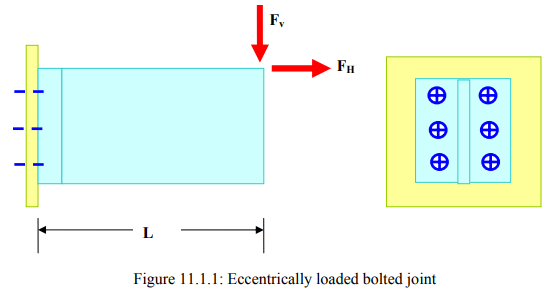It is easy to note that the tension in the screws cannot be obtained by equations of statics alone. Hence, additional equations must be formed to solve for the unknowns for this statically indeterminate problem. Since there is a tendency for the bracket to rotate about point O then, assuming the bracket to be rigid, the following equations are easily obtained.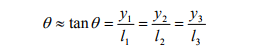where yi =elongation of the i-th bolt

li=distance of the axis of the i-th bolt from point O.

If the bolts are made of same material and have same dimension, then

fi = kyi

where fi =force in the i-th bolt

k =stiffness of the bolts

Thus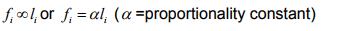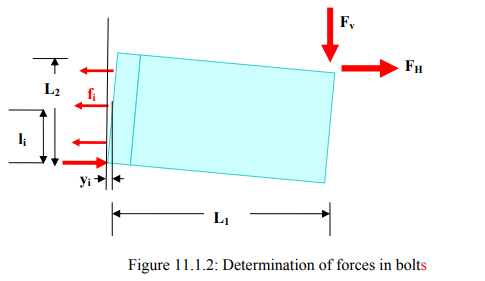Using the moment balance equations about O, the lowermost point in the left side, the following equation is obtained.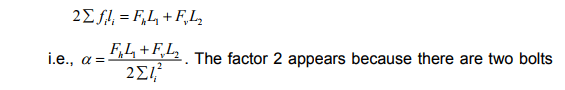in a row.

Thus the force in the i-th screw is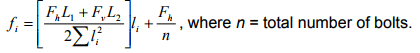For safe design of the joint it is therefore required that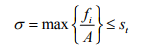where St =allowable tensile stress of the bolt.

Note that Fvcauses also direct shear in the bolt. Its effect may be ignored for a preliminary design calculation.

Consider, now, a bracket, which carries a vertical load F . The bracket, in this case, is connected to the wall by four rivets as shown in figure 11.1.2. The force,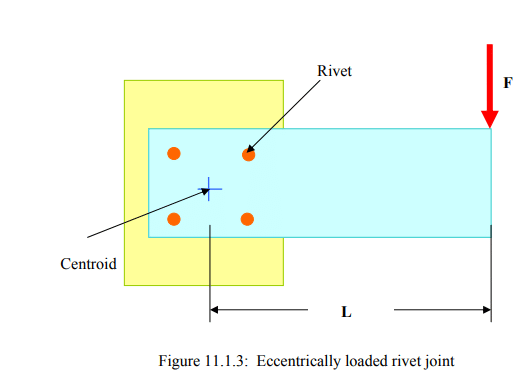in addition to inducing direct shear of magnitude  F/4 in each rivet, causes the whole assembly to rotate. Hence additional shear forces appear in the rivets. F Centroid Rivet L Figure 11.1.3: Eccentrically loaded rivet joint

Once again, the problem is a statically indeterminate one and additional assumptions are required. These are as following:

(i) magnitude of additional shear force is proportional to the distance between the rivet center and the centroid of the rivet assembly, whose coordinates are defined as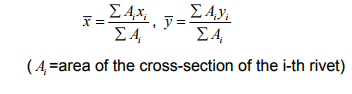(ii) directions of the force is perpendicular to the line joining centroid of the rivet group and the rivet center and the sense is governed by the rotation of the bracket.

Noting that for identical rivets the centroid is the geometric center of the rectangle, the force in the i-th rivet is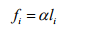where α =proportional constant

li=distance of the i-th rivet from centroid.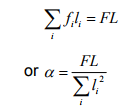Thus, the additional force is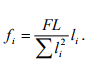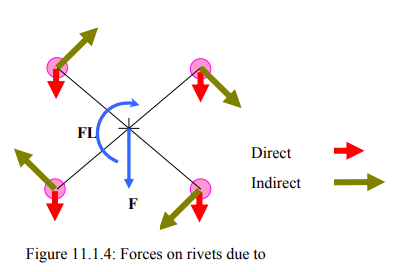The net force in the i-th rivet is obtained by parallelogram law of vector addition as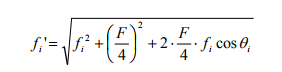where θ i =angle between the lines of action of the forces shown in the figure.

For safe designing we must have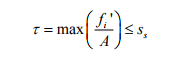where ss  =allowable shear stress of the rivet.

The document Design of Eccentrically Loaded Bolted/Riveted Joints Notes | Study Design of Machine Elements - Mechanical Engineering is a part of the Mechanical Engineering Course Design of Machine Elements.
All you need of Mechanical Engineering at this link: Mechanical EngineeringUse Code STAYHOME200 and get INR 200 additional OFF

### Up next## Design of Machine Elements

51 videos|63 docs|83 tests

### Up nextTrack your progress, build streaks, highlight & save important lessons and more!

,

,

,

,

,

,

,

,

,

,

,

,

,

,

,

,

,

,

,

,

,

;